Reach Us+44-1522-440391
Optimization of Microstructure Optical Fiber using PWE Method for Investigation of Glucose in Intralipid | OMICS International
Journal of Lasers, Optics & Photonics
All submissions of the EM system will be redirected to Online Manuscript Submission System. Authors are requested to submit articles directly to Online Manuscript Submission System of respective journal.

# Optimization of Microstructure Optical Fiber using PWE Method for Investigation of Glucose in Intralipid

Palai G*, Dalai PK, Kumar A, Satpathy A, Kushwaha JK, Jha A and Panda A

Gandhi Institute for Technological Advancement (GITA), Bhubaneswar, India

*Corresponding Author:
Palai G
Gandhi Institute for Technological
Advancement (GITA) Bhubaneswar, India
Tel: +919439045946
Fax: 0674-2538661
E-mail: [email protected]

Received Date: April 04, 2015 Accepted Date: April 28, 2015 Published Date: April 30, 2015

Citation: Palai G, Dalai PK, Kumar A, Satpathy A, Kushwaha JK, et al. (2015) Optimization of Microstructure Optical Fiber using PWE Method for Investigation of Glucose in Intralipid. J Laser Opt Photonics 2:117. doi:10.4172/2469-410X.1000117

Copyright: © 2015 Palai G, et al. This is an open-access article distributed under the terms of the Creative Commons Attribution License, which permits unrestricted use, distribution, and reproduction in any medium, provided the original author and source are credited.

Visit for more related articles at Journal of Lasers, Optics & Photonics

#### Abstract

Investigation of glucose in intralipid using microstructure optical fiber is reported in this paper. The optical fiber is realized by 5 × 5 air holes on square lattice silicon substrate. The principle of measurement of glucose in intralipid is based on the variation of electric field with respect to percentage of glucose. The simulation for electric field distribution is done using plane wave expansion method, where optimization is realized by varying radius of air holes and lattice spacing of crystal structure. With varying both radius and lattice spacing and employing PWE method, simulation result revealed that both peak electric field and output power emerging from microstructure fiber increases with the increase of glucose concentration for certain radii and lattice spacing. Aside this present result divulged that microstructure fiber with lattice spacing of 1 μm and radius of air holes of 300 nm is suitable to investigate glucose in an intralipid. Finally an experimental setup is proposed to measure the glucose concentration.

#### Keywords

Microstructure fiber; Plane wave expansion; Intralipid

#### Introduction

As far as optical technology scenario is concerned, standard/ conventional optical fiber plays an important role to make different applications in the field of photonics. The concept of standard optical fiber has been originated since long back. The transportation of light through conventional fiber is based on the principle of total internal reflection . The principle Total Internal Reflection (TIR) is accomplished, if the refractive index of core is higher than cladding, otherwise TIR phenomenon cannot be achieved. However a new kind of optical fiber is invention just before 18 years . The new class of optical fiber does not keep constrain regarding higher or lower refractive index between core and cladding. Depending on the lower and higher refractive index of core with respect to cladding, this optical fiber is divided in to two groups such as microstructure fiber and photonic crystal fiber. Light transports through microstructure govern the principle of Modified Total Internal Reflection (M-TIR), where M-TIR is analogous to TIR from standard optical fiber. It relies on a high index core region effective index provided by the microstructure region, where photonic crystal fiber is governed by the principle of photonic band gap. The physical mechanisms of photonic crystal fiber are different from M-TIR guiding fiber. The concept of photonic band gap is understood by light at certain wavelength regime cannot propagate through optical fiber . Several types of application are employed using microstructure optical fiber in the field of photonics. We in this paper have used a MSOF for sensing application. Microstructure Optical Fiber (MSOF) is a new kind of optical fiber which has unique properties that offers an opportunity to manipulate light in new ways . It provides a new platform for novel class of all photonic devices. The transportation of light energy through microstructure fiber depends on its principle, which is governed by modified total internal reflection. As far as sensing applications using photonic devices are concerned, different types of works have appeared in literature [5-9]. In above references, photonic crystal structure are used to investigate different concentrations, for example reference  determines the concentration of sugar, salt and alcohol in their aqueous solution using two dimensional photonic crystal structure where percentage of sucrose in PAM-Hydrogel solution is investigated in reference  and also strength of Cygel is investigated using same technique . Aside these, glycerol and hemoglobin in human blood are measured using 3D photonic crystal structure in reference  and  respectively. Apart from this, using similar type of microstructure fiber the concentration of potassium chloride solution is obtained in reference . There is a drawback divulged in this case, for example total electric field emerging from optical fiber is not cogitated for finding out the percentage of potassium chloride solution. In this case electric field distribution at particular point is considered which leads to difficulty for setting the photo detector. But we in this paper consider total electric field coming out from optical fiber with respect to different concentration of glucose in an intralipid.

As far as importance of intralipid is concerned, a diabetic metabolic control plays an important role for human body now a days. Both hypo and hyper glycemia diseases are dangerous for us. To maintain the normalcy of glucose in human blood, the consumption of intralipid is essential for affected people. Keeping the view of importance of glucose in an intralipid, we propose a device which measures the concentration of glucose in an intralipid.

This paper is organized as follows; structure analysis and optimization technique is discussed in section 2. Mathematical approach for plane wave expansion is given in section 3. Results and discussion is interpreted in section 4. Experimental setup is proposed in section 5 and conclusions are drawn in section 6.

#### Structure Analysis and Optimization Technique

For the sake of measurement of glucose in intralipid, a microstructure fiber with square lattice of 5 × 5 air holes with defect at center is cogitated here, which is shown in (Figure 1).

Figure 1: schematic diagram of microstructure fiber having diameter ‘d’ and lattice spacing of a=1 μm.

In Figure 1, it is seen that silicon is background material and 5 × 5 air holes are etched on the substrate except at centre. The centre is treated as core, where air holes are treated as cladding. When light incidents on this structure then light transports through fiber based on the principle of modified total internal reflection. As this paper concentrates on the measurement of glucose in an intralipid, intralipid having different percentage of glucose is infiltrated into air holes. As far as principle of measurement is concerned it depends on the variation of both peak electric field and output power with respect to glucose concentration. In this case light having wavelength 589 nm is incident on said microstructure fiber containing different percentage of glucose in an intralipid then electric field distribution through optical fiber is measured. Since the percentage of glucose concentration in an intralipid is different, the output electric fields as well as power are different for different glucose concentration. As far as optimization technique is concerned, here we use plane wave expansion to find out transmitted electric field through MSOF. Using PWE method we have done simulation taking different values of lattice spacing (a) and radius of air holes (r). Here intralipid with different percentages of glucose concentrations are infiltrated in the micro structure fiber. Almost in all cases, the variation of electric field as well as power emerging from fiber is found nonlinear with respect to glucose concentration in an intralipid which ranges from 1 mg/dL to 70 mg/dL. To realize a sensing application in the same we are interested to obtain linear variation of electric field and power with respect to glucose concentration by changing different ‘r’ and ‘a’. So by the method of hit and trail basis (varying r and a), we obtained the same linear variation at certain values of ‘r’ and ‘a’. To do so, here we have done simulation taking different value of ‘r’ and ‘a’. The simulation result for r=200 nm, 300 nm and 450 nm at lattice constant of 1 μm is shown, which is described in simulation and discussion section.

#### Mathematical approach

To find out electric field distribution n microstructure fiber, we use following Maxwell’s four generalized equations and solving these equations we obtain the Helmholtz equation, which is given by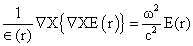(1)

The reason for choosing Helmholtz equation is that this equation gives the solution for electric field distribution through microstructure fiber.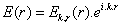(2)

Where Ek,ris the periodic function with periodicity of lattice.

The wave functions are represented in terms of Bloch wave and expanded in to Fourier series over lattice vector, which is expressed as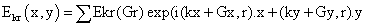(3)

Here E’kr(Gr) are Eigen vectors to be found during the Eigen problem solution. Gx and Gy are called Fourier coefficients for harmonics. Using above equations, we solved Eigen value problem and compute the field distribution in the microstructure optical fiber.

From above field distribution, the peak electric field corresponding to each field distribution is computed. Since we want to investigate the power through optical fiber at different concentrations of glucose in an initial lipid, then we consider the equation which gives the relationship between power electric filed. And the same expression is collected from the literature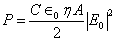(4)

Where ‘C’ is velocity of light,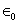is permittivity of free space,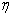is the refractive index modulation, ‘A’ is the area of the square lattice,is the peak electric field.

#### Result and Discussion

To find out the percentage of glucose concentration in intralipid we use micro structure optical fiber having silicon as background material with 5 × 5 air holes on square lattice structure with defect at center and the simulation for electric field distribution is made by employing plane wave expansion method . The transportation of light energy through fiber depends on the principle of modified total internal reflection. The M-TIR is realized by lower refractive index (air filled region) on the substrate having higher refractive index in microstructure fiber. To find out such electric field distribution, we choose different values of radius and lattice spacing (‘r’ and ‘a’). In all values of r and a, the non-linear variation of peak electric field (emerging from fiber) with respect to concentration of glucose in intralipid is found. To envisage a linear variation for sensing application, we tried to choose different radius and lattice spacing where peak electric field varies linearly with respect to concentration of glucose in intralipid. Though we have made simulation for different values of ‘r’ and ‘a’, here we have showed three simulation results which is mentioned in (Figures 2a-2c) for radius 200 nm, 300 nm and 450 nm respectively.

Figure 2a: electric field distribution in microstructure optical fiber with radius of air holes of (r), 200 nm containing 1 mg/dL of glucose in intralipid and lattice spacing (a) of 1 μm.

Figure 2b: electric field distribution in microstructure optical fiber with radius of air holes of (r), 300 nm containing 1 mg/dL of glucose in intralipid and lattice spacing (a) of 1 μm.

Figure 2c: electric field distribution in microstructure optical fiber with radius of air holes of (r), 450 nm containing 1 mg/dL of glucose in intralipid and lattice spacing (a) of 1 μm.

Figures 2a-2c represent the electric field distribution in above mentioned optical fiber with respect to radius of air holes, of 200 nm, 300 nm and 450 nm at lattice spacing of 1 μm respectively. In these figures, electric field (V/μm) is taken along z-axis, where length and breadth is taken along x and y axis respectively. Aside this, from above graphs it is seen that no propagation is found at the center of the optical fiber due to defect is made at the same. Again it is observed that definite electric field distribution is found at different locations in the microstructure fiber. Since the transportation of light is governed M-TIR, the magnitude of electric field distribution is differed from different region in the fiber. The electric field corresponding to different co-ordinates in the same fiber is computed and then total electric field at different radius of air holes contains different percentages of glucose concentration are obtained. Using above concept and deploying equation (4), the power transmitting through optical fiber is computed for different radius and lattice spacing with respect to glucose concentration in intralipid. The result for the same is shown in (Figure 3).

Figure 3 represents the variation of output power in mW is taken along y-axis, where the concentration of glucose varies from 1 mg/dL to 70 mg/dL along x-axis. From Figure 3, it is seen that red colour variation represents transmitted power for r=200 nm and blue colour variation represents transmitted power for r=300 nm, where green colour variation represents transmitted power for r=450 nm at constant a=1 μm. It is seen from Figure 3 that nonlinear variation of output power is found for both radius of 200 nm (red colour) and 450 nm (green colour), For an example output power decreases from 6.802 mW to 0.856 mW with glucose concentration increases from 1 mg/dL to 50 mg/dL and then increases to 6.013 mW at concentration of 70 mg/dL for radius 200 nm. Similarly it is observed that output power suddenly increases from 3.773 mW to 12.242 mW with glucose concentration increases from 1 mg/dL to 10 mg/dL and further decreases to 2.781 mW at 50 mg/dL and then it increases to 6.013 mW at concentration, 70 mg/dL. These random variations do not support to carry out sensing application, however different nature of variation is observed, when we choose radius of air holes of 200 nm, for example output power from such structure increases from 2.311 mW to 9.276 mW with the increase of glucose concentration, which ranges from 1 mg/dL to 70 mg/dL. This linear variation (increasing of power with concentration) leads to sensing application for measurement of glucose concentration. From the above variation (power increases with increasing glucose concentration), it is realized that the glucose concentration can be estimated by knowing transmitted power. Aside this it is also observed that the variation of transmitted power with respect to glucose concentration is fitted nicely with linearship (R2 =0.998) which is inset in Figure 3. This excellent linear variation leads to an accurate investigation of glucose concentration in intralipid from range 10 mg/dL to 50 mg/dL.

Though we have shown these three values only (r=200 nm, 300 nm and 450 nm) for a=1 μm in this paper but we have done simulation for different values of a and r. In these cases, the nonlinear variation of both electrified and power are found. Although the liner variation of power is found at r=300 nm and a=1 μm, the reason for choosing r=200 nm and 450 nm is that these are considered as example and comparative study with r=300 nm.

#### Proposed Experimental Setup

From simulation and discussion section, it is realized that the concentration of glucose in intralipid can be investigated by using microstructure fiber having radius of air holes of 300 nm and lattice spacing of 1 μm by knowing transmitted power emerging from microstructure fiber. The same measurement can by realized by using following photonic device.

Figure 4 represents the experimental setup to measure the glucose concentration using microstructure fiber having radius of air holes of 300 nm with lattice spacing of 1 μm. In this figure, it is seen that sodium light having wavelength, 589 nm emanating from source and incident on microstructure fiber containing different percentage of glucose concentration in air holes, then emerging light having definite power (mW) is recorded at output end and finally measured by power meter. Using above principle, the emerging power from optical fiber is measured with respect to different percentage of glucose in intralipid which is infiltrated in MSOF. Since different percentage of glucose concentration in an optical fiber gives different field distribution, the output power differed from different concentrations of glucose.

#### Conclusions

Optimization of microstructure fiber for estimation of glucose in an intralipid is thoroughly explored in this paper. The optimization of the same is made by employing plane wave expansion method with the variation of both radius of air holes and lattice spacing of the structure. The simulation results revealed that the nature of variation of both electric field and transmitted power depends on radius and lattice spacing including structure and configuration. Also an experimental setup is designed to investigate the same glucose concentration. Finally present simulation result divulged that output power varies linearly with respect to glucose concentration for radius of air holes of 300 nm at lattice constant of 1 μm and also it is found that this structure can be a good candidate to measure the concentration of glucose in intralipid from 10 mg/dL to 50 mg/dL accurately.

#### References

Select your language of interest to view the total content in your interested language

### Article Usage

• Total views: 12322
• [From(publication date):
July-2015 - Aug 18, 2019]
• Breakdown by view type
• HTML page views : 8531
• PDF downloads : 3791

## Post your commentCan't read the image? click here to refresh
###### Peer Reviewed Journals

Make the best use of Scientific Research and information from our 700 + peer reviewed, Open Access Journals

International Conferences 2019-20

Meet Inspiring Speakers and Experts at our 3000+ Global Annual Meetings

Top